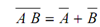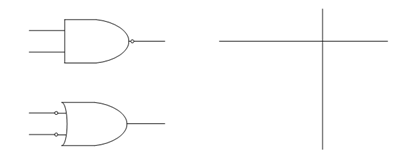## De Morgans Second Theorem Assignment Help

Assignment Help: >> De Morgans Theorems - De Morgans Second Theorem

De Morgan's Second Theorem:

It says that complement of product equals the summation of complements.The LHS of the equation explain a NAND (NOT-AND) gate and the RHS of the equation explain an OR gate with inverted inputs and both of the equations have the same truth table as illustrated in FigureFigure: (a) NAND Logic Gate, (b) OR Gate with Inverted Inputs, and (c) Truth Table